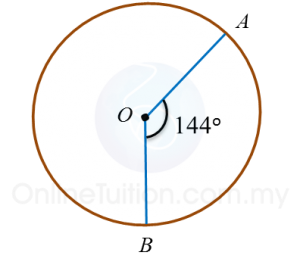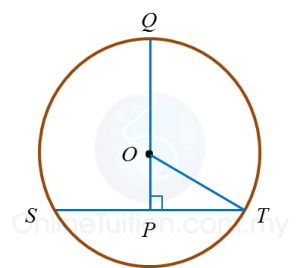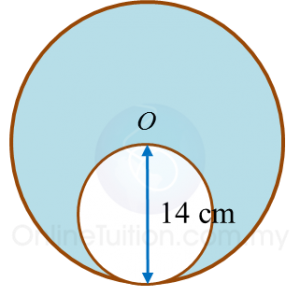# 5.2.1 Circles, PT3 Focus Practice

5.2.1 Circles, PT3 Focus Practice

Question 1:
Diagram below shows a circle with centre O.The radius of the circle is 35 cm.
Calculate the length, in cm, of the major arc AB.

Solution:
Angle of the major arc AB = 360o – 144o= 216o
$\begin{array}{l}\text{Length of major arc}AB\\ =\frac{{216}^{o}}{{360}^{o}}×2\pi r\\ =\frac{{216}^{o}}{{360}^{o}}×2×\frac{22}{7}×35\\ =132\text{cm}\end{array}$

Question 2:
In diagram below, O is the centre of the circle. SPQ and POQ are straight lines.The length of PO is 8 cm and the length of POQ is 18 cm.
Calculate the length, in cm, of SPT.

Solution
:
Radius = 18 – 8 = 10 cm
PT2 = 102 – 82
= 100 – 64
= 36
PT = 6 cm
Length of SPT = 6 + 6
= 12 cm

Question 3:
Diagram below shows two circles. The bigger circle has a radius of 14 cm with its centre at O.
The smaller circle passes through O and touches the bigger circle.Calculate the area of the shaded region.

Solution:

### 2 thoughts on “5.2.1 Circles, PT3 Focus Practice”

1.for question 5, may i know why KO,ON & OP = 7 cm? wasnt it supposed to be 14 cm ?

•Thanks for pointing out our mistake.